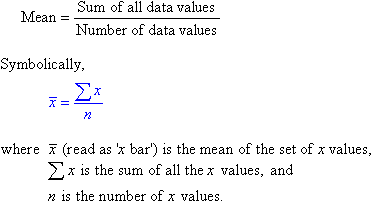# Java Program To Calculate Average Of N Numbers

Java program to calculate the average of N numbers. Here is the code to calculate the average of N numbers or average of 2 or 3 numbers. The following code has been written in three different ways, using standard values, using do while, recursion, command line arguments, creating a separate class, user-defined method.

How to calculate the average of N Numbers?

The calculation of the average of N numbers ins quite simple: Here is the formula for it. Hope you get to understand-The Average has been calculated as the sum of all data values / Number of data values.

The following program can be applied for either average of two numbers or average of three numbers, or an average of N numbers. In the case of all N number. Just replace the SOP with the above-given formula.

The following code has been written in five different ways for both programs along with the sample output for an average of 2 numbers and average of three numbers or N numbers, in using standard values , while loop, for loop, do while loop, command line arguments, user define method, creating a separate class.

1. Java Program Calculate Average Using Standard values

There you go, the basic universal syntax for the average of N Numbers program or given 2 or 3 numbers as you wish.

output:

2. Taking inputs through scanner class

Java code for obtaining an average of numbers taking inputs through scanner class.

output:

3. Using Command Line Arguments

By using command line arguments: If you have no idea about command line arguments then do check out our guide here – what are command line arguments in java with examples.

output:

4. Using the User Defined Method

By using the user-defined method. You can do allocate whatever method. This is just for an example program.

output:

5. Through creating a separate class

Java program for getting  average of numbers through creating a separate class( ) and taking inputs through scanner class

Share your ideas and thoughts for the above program.

#Happy Coding#

x

## X Star Pattern Java Program – Patterns

Java program to print X star pattern program – We have written the below print/draw ...